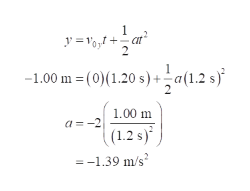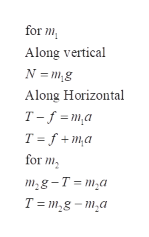Question
4 views

Objects with masses m1 = 10.0 kg and m2= 5.00 kg are
connected by a light string that passes over a frictionless pulley
as in Figure P4.64. If, when the system starts from rest, m 2 falls
1.00 m in 1.20 s, determine the coefficient of kinetic friction
between m 1 and the table.

check_circle

Step 1

Use the kinematics equation of position in terms of acceleration, in order to find the acceleration of the mass m2.help_outlineImage Transcriptionclose-at y =V,1 + -1.00 m = (0)(1.20 s) +a(1.2 s) 1.00 m a = -2 (1.2 s)* = -1.39 m/s fullscreen
Step 2

In the above equation the negative sign of the acceleration shows that the acceleration is towards downward.

Draw the free body diagram of the objects are:

For mass m1 and m2 are shown below:

Step 3

Write the force equation...help_outlineImage Transcriptionclosefor m1, Along vertical N = m,g Along Horizontal T-f =m,a T = f + m,a for m, mg-T = m,a T = m,g – m,a fullscreen

### Want to see the full answer?

See Solution

#### Want to see this answer and more?

Solutions are written by subject experts who are available 24/7. Questions are typically answered within 1 hour.*

See Solution
*Response times may vary by subject and question.
Tagged in

### Newtons Laws of Motion